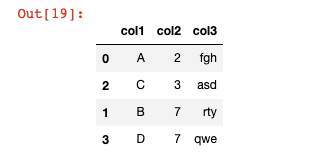# Common Operations in Pandas

Hey - Nick here! This page is a free excerpt from my \$199 course Python for Finance, which is 50% off for the next 50 students.

This lesson will explore common operations in the pandas Python library. The purpose of this lesson is to explore important pandas operations that have not fit into any of the sections we've discussed so far.

## The DataFrame We Will Use In This Lecture

I will be using the following DataFrame in this section:

``````df = pd.DataFrame({'col1':['A','B','C','D'],

'col2':[2,7,3,7],

'col3':['fgh','rty','asd','qwe']})``````

## How To Find Unique Values in a Pandas Series

Pandas has an excellent method called `unique` that can be used to find unique values within a pandas Series. Note that this method only works on Series and not on DataFrames. If you try to apply this method to a DataFrame, you will encounter an error:

``````df.unique()

#Returns AttributeError: 'DataFrame' object has no attribute 'unique'``````

However, since the columns of a pandas DataFrame are each a Series, we can apply the `unique` method to a specific column, like this:

``````df['col2'].unique()

#Returns array([2, 7, 3])``````

Pandas also has a separate `nunique` method that counts the number of unique values in a Series and returns that value as an integer. For example:

``````df['col2'].nunique()

#Returns 3``````

Interestingly, the `nunique` method is exactly the same as `len(unique())` but it is a common enough operation that the pandas community decided to create a specific method for this use case.

## How To Count The Occurence of Each Value In A Pandas Series

Pandas has a function called `counts_value` that allows you to easily count the number of time each observation occurs. An example is below:

``````df['col2'].value_counts()

"""

Returns:

7    2

2    1

3    1

Name: col2, dtype: int64

"""``````

## How To Use The Pandas `apply` Method

The `apply` method is one of the most powerful methods available in the pandas library. It allows you to apply a custom function to every element of a pandas Series.

As an example, imagine that we had the following function `exponentify` that takes in an integer and raises it to the power of itself:

``````def exponentify(x):

return x**x``````

The `apply` method allows you to easily apply the `exponentify` function to each element of the Series:

``````df['col2'].apply(exponentify)

"""

Returns:

0         4

1    823543

2        27

3    823543

Name: col2, dtype: int64

"""``````

The `apply` method can also be used with built-in functions like `len` (although it is definitely more powerful when used with custom functions). An example of the `len` function being used in conjunction with `apply` is below:

``````df['col3'].apply(len)

"""

Returns

0    3

1    3

2    3

3    3

Name: col3, dtype: int64

"""``````

## How To Sort A Pandas DataFrame

You can filter a pandas DataFrame by the values of a particular column using the `sort_values` method. As an example, if you wanted to sort by `col2` in our DataFrame `df`, you would run the following command:

``df.sort_values('col2')``

The output of this command is below:There are two things to note from this output:

1. As you can see, each row preserves its index, which means the index is now out of order.
2. As with the other DataFrame methods, this does not actually modify the original DataFrame unless you force it to using the `=` assignment operator or by passing in `inplace = True`.

## Moving On

In this lesson, we explored various elements of the pandas library that did not fit into the other lessons of this course. After a brief round of practice problems, we will discuss data input and output in pandas.